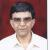true

Learn Python Training from the Best Tutors

•Affordable fees
•1-1 or Group class
•Flexible Timings
•Verified Tutors

Search in

# A program to calculate Correlation CoefficientAshish K Sharma
23 Jun 00

Task: Calculating the Correlation-coefficient using Python

We know that the correlation coefficient is calculated using the formula

nΣxy- ΣxΣy / (√(nΣx^2-(Σx)^2) * (nΣy^2-(Σy)^2))

In the above formula, n is the total number of values present in each set

of numbers (the sets have to be of equal length). The two sets of numbers

are denoted by x and y (it doesn’t matter which one you denote as which).

The other terms are described as follows:

Σxy :Sum of the products of the individual elements of the two sets

of numbers, x and y

Σx : Sum of the numbers in set x

Σy:  Sum of the numbers in set y

Σx^2:Square of the sum of the numbers in set x

Σy^2:Square of the sum of the numbers in set y

(Σx)^2:Sum of the squares of the numbers in set x

(Σy)^2:Sum of the squares of the numbers in set y/

Let us now write a Python Program which calculates the correlation coefficient for us. We will be using the following two functions in the program:

1. Sum(x) : Using this function on a list of numbers,x will sum up the numbers in the list.
1. Zip(x,y): returns the list of corresponding numbers in lists x,y which  you can then use in a loop to perform other operations.

import os
import sys
#A Program to calculate the correlation coefficient

def find_corr_x_y(x,y):
n = len(x)

#Find the sum of the products
prod = []
for xi,yi in zip(x,y):
prod.append(xi*yi)

sum_prod_x_y = sum(prod)
sum_x = sum(x)
sum_y = sum(y)

squared_sum_x = sum_x ** 2
squared_sum_y = sum_y ** 2

x_square = []

for xi in x:
x_square.append(xi**2)

x_square_sum = sum(x_square)

y_square = []
for yi in y:
y_square.append(yi**2)

y_square_sum = sum(y_square)

numerator = n * sum_prod_x_y - sum_x * sum_y
dterm1 = n*x_square_sum - squared_sum_x
dterm2 = n*y_square_sum - squared_sum_y
denm = (dterm1 *dterm2) ** 0.5
corr = numerator / denm
return corr

crr = 0

X1 = [5.1,3.2,3,1.4,3.8,1.0,2.8,-0.3,6.9,2.5,6.2,4.6]
Y = [30,29,30,35,36,36,34,48,24,27,21,30]

if (len(X1) == len(Y)):
crr = find_corr_x_y(X1,Y)
print("Pearson product-moment Correlation Coefficient = {0}".format(crr))

if (crr >= 0.8):
print("Strong Positive Correlation")

elif (crr <= -0.8):
print("Strong Negative Correlation")
else:
print("Sorry,the data set lengths are not equal")

The find_corr_x_y() function accepts two arguments, x and y, which are the two sets of numbers we want to calculate the correlation for. Inside this function all terms used for calculating the Correlation coefficient are obtained. Also, correlation coefficient is only calculated when the list of numbers passed to the function are equal in length.

OUTPUT

>>>

Pearson product-moment Correlation Coefficient = -0.823545657378

Strong Negative Correlation

>>>

Try writing this program,students, in your computer and see how it runs,with equal and unequal lists of numbers.

0 Dislike
Follow 1## Other Lessons for You

File Handling in Python - Basic Concept
File (Flat) Handling in Python Types of files in python: 1: Text file: Stores data in the form of characters. Customarily used to store text/string data. 2: Binary file: Stores data in the form of bytes....DBMS - SQL - Any/All
All - Operator SELECT empno, sal FROM emp WHERE sal > ALL (1999, 2999, 3999); Output of Above query is same as below query SELECT empno, sal FROM emp WHERE sal > 1999 AND sal > 2999...Write your first Python program in 10 minutesCode: Gantt Chart: Horizontal bar using matplotlib for tasks with Start Time and End Time
import pandas as pd from datetime import datetimeimport matplotlib.dates as datesimport matplotlib.pyplot as plt def gantt_chart(df_phase): # Now convert them to matplotlib's internal format... ...### Looking for Python Training classes?

Learn from Best Tutors on UrbanPro.

Are you a Tutor or Training Institute?

Join UrbanPro Today to find students near you
X

### Looking for Python Training Classes?

The best tutors for Python Training Classes are on UrbanPro

• Select the best Tutor
• Book & Attend a Free Demo
• Pay and start Learning### Learn Python Training with the Best Tutors

The best Tutors for Python Training Classes are on UrbanProUrbanPro.com is India's largest network of most trusted tutors and institutes. Over 55 lakh students rely on UrbanPro.com, to fulfill their learning requirements across 1,000+ categories. Using UrbanPro.com, parents, and students can compare multiple Tutors and Institutes and choose the one that best suits their requirements. More than 7.5 lakh verified Tutors and Institutes are helping millions of students every day and growing their tutoring business on UrbanPro.com. Whether you are looking for a tutor to learn mathematics, a German language trainer to brush up your German language skills or an institute to upgrade your IT skills, we have got the best selection of Tutors and Training Institutes for you. Read more